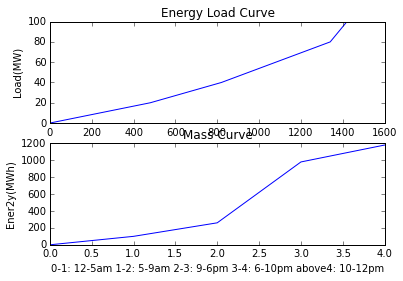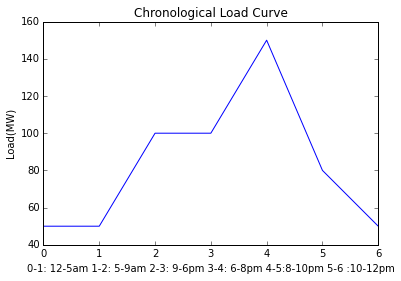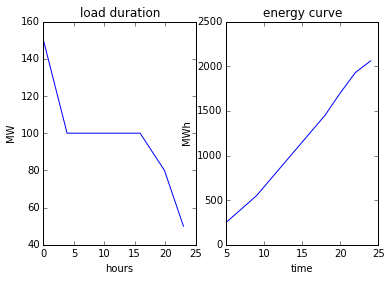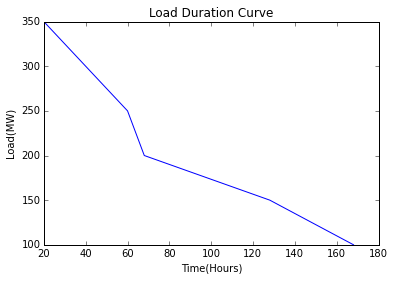# Ch-1 : Introduction¶

## exa 1.1 page 1¶

In :
from __future__ import division
import numpy as np
B=100 #W(8Bulb)
F=60 #W(2Fan)
L=100 #W(2Light)
print "(a) Connected Load = %0.2f W "%LoadConnected
#12 midnight to 5am
demand1=1*F #W
#5am to 7am
demand2=2*F+1*L #W
#7am to 9am
demand3=0 #W
#9am to 6pm
demand4=2*F #W
#6pm to midnight
demand5=2*F+4*B #W
DEMAND=np.array([demand1,demand2, demand3, demand4, demand5])
max_demand=max(DEMAND)
print "(b) Maximum demand = %0.2f W "%max_demand
print "(c) Demand factor = %0.3f"%df
E=demand1*5+demand2*2+demand3*2+demand4*9+demand5*6 #Wh
E=E/1000 #kWh
print "(d) Energy consumed during 24 hours = %0.2f kWh "%E
print "(e) Energy consumed during 24 hours if all devices are used = %0.2f kWh"%Edash

(a) Connected Load = 1120.00 W
(b) Maximum demand = 520.00 W
(c) Demand factor = 0.464
(d) Energy consumed during 24 hours = 4.94 kWh
(e) Energy consumed during 24 hours if all devices are used = 26.88 kWh


## exa 1.2 page 3¶

In :
import numpy as np
#12 midnight to 5am
d1A=100 #W
#5am to 6am
d2A=1.1*1000 #W
#6am to 8am
d3A=200 #W
#8am to 5pm
d4A=0 #W
#5pm to 12 midnight
d5A=500 #W
#11 pm to 7am
d1B=0 #W
#7 am to 8 am
d2B=300 #W
#8 am to 10 am
d3B=1*1000 #W
#10 am to 6 pm
d4B=200 #W
#6 pm to 11 pm
d5B=600 #W
DEMAND_A=np.array([d1A, d2A, d3A, d4A, d5A]) #W
DEMAND_B=np.array([d1B, d2B, d3B, d4B, d5B]) #W
max_demand_A=max(DEMAND_A) #W
max_demand_B=max(DEMAND_B) #W
print "Demand factor of consumer A & B are : %0.2f & %0.2f"%(df_A,df_B)
gd_factor=(max_demand_A+max_demand_B)/max_demand_A
print "Group diversity factor = %0.3f"%gd_factor
E_A=d1A*5+d2A*1+d3A*2+d4A*9+d5A*7 #Wh
E_B=d1B*8+d2B*1+d3B*2+d4B*8+d5B*5 #Wh
E_A=E_A/1000 #kWh
E_B=E_B/1000 #kWh
print "Energy consumed by A & B during 24 hours = %0.2f & %0.2f kWh "%(E_A,E_B)
Emax_A=max_demand_A*24/1000 #kWh
Emax_B=max_demand_B*24/1000 #kWh
print "Maximum energy consumer A & B can consume during 24 hours = %0.2f & %0.2f kWh "%(Emax_A,Emax_B)
ratio_A=E_A/Emax_A
ratio_B=E_B/Emax_B
print "Ratio of actual energy to maximum energy of consumer A & B : %0.4f & %0.4f"%(ratio_A,ratio_B)

Demand factor of consumer A & B are : 0.44 & 0.33
Group diversity factor = 1.909
Energy consumed by A & B during 24 hours = 5.50 & 6.90 kWh
Maximum energy consumer A & B can consume during 24 hours = 26.40 & 24.00 kWh
Ratio of actual energy to maximum energy of consumer A & B : 0.2083 & 0.2875


## exa 1.3 page 6¶

In :
n1=600 #No. of apartments
L1=5 #kW#Each Apartment Load
n2=20 #No. of general purpose shops
L2=2 #kW#Each Shop Load
df=0.8 #demand factor
#1 Floor mill
df3=0.7 #demand factor
#1 Saw mill
df4=0.8 #demand factor
#1 Laundry
df5=0.65 #demand factor
#1 Cinema
df6=0.5 #demand factor
#Street lights
n7=200 #no. of tube lights
L7=40 #W#Load of each light
df8=0.5 #demand factor
gdf_r=3 #group diversity factor
pdf_r=1.25 #peak diversity factor
gdf_c=2 #group diversity factor
pdf_c=1.6 #peak diversity factor
#Solution :
#Maximum demand of each apartment
dmax_1a=L1*df8 #kW
#Maximum demand of 600 apartment
dmax_a=n1*dmax_1a/gdf_r #kW
#demand of apartments at system peak time
d_a_sp=dmax_a/pdf_r #kW
#Maximum Commercial demand
dmax_c=(n2*L2*df+L3*df3+L4*df4+L5*df5+L6*df6)/gdf_c #kW
#Commercial demand at system peak time
d_c_sp=dmax_c/pdf_c #kW
#demand of street light at system peak time
d_sl_sp=n7*L7/1000 #kW
#Increase in system peak demand
DI=d_a_sp+d_c_sp+d_sl_sp #kW
print "Increase in system peak demand  %0.2f kW" %DI,

Increase in system peak demand  438.00 kW


## exa 1.4 page 12¶

In :
#12 to 5 am
L1=20 #MW
t1=5 #hours
#5 to 9 am
L2=40 #MW
t2=4 #hours
#9 to 6 pm
L3=80 #MW
t3=9 #hours
#6 to 10 pm
L4=100 #MW
t4=4 #hours
#10 to 12 am
L5=20 #MW
t5=2 #hours
#Energy Poduced in 24 hours
E=L1*t1+L2*t2+L3*t3+L4*t4+L5*t5 #MWh
print "Energy Supplied by the plant in 24 hours = %0.2f MWh" %E
print "Load Factor = %0.2f %% "%LF

Energy Supplied by the plant in 24 hours = 1420.00 MWh
Load Factor = 59.17 %


## exa 1.5 page 13¶

In :
from __future__ import division
C=125 #MW#Installed Capacity
#12 to 5 am
L1=20 #MW
t1=5 #hours
#5 to 9 am
L2=40 #MW
t2=4 #hours
#9 to 6 pm
L3=80 #MW
t3=9 #hours
#6 to 10 pm
L4=100 #MW
t4=4 #hours
#10 to 12 am
L5=20 #MW
t5=2 #hours
#Energy Poduced in 24 hours
E=L1*t1+L2*t2+L3*t3+L4*t4+L5*t5 #MWh
CF=LF/C #%#Capacity Factor
print "Capacity Factor = %0.3f" %CF
UF=100/C #%#Utilisation Factor
print "Utilisation Factor = %.1f" %UF

Capacity Factor = 0.473
Utilisation Factor = 0.8


## exa 1.6 page 13¶

In :
%matplotlib inline
from matplotlib.pyplot import plot, show, title, xlabel, ylabel, subplot
from numpy import array
import numpy as np
#12 to 5 am & 10 to 12 am
L1=20 #MW
E1=L1*24 #MWh
#5 to 9 am
L2=40 #MW
E2=E1+(L2-L1)*17 #MWh
#9 to 6 pm
L3=80 #MW
E3=E2+(L3-L2)*13 #MWh
#6 to 10 pm
L4=100 #MW
E4=E3+(L4-L3)*4 #MWh
#Plotting Energy load curve
L=np.array([0,L1,L2,L3,L4]) #MW
E=np.array([0,E1,E2,E3,E4]) #Mwh
subplot(2,1,1)
plot(E,L)
xlabel('Energy(MWh)')
#Energy Supplied
#Upto 5am
t1=5 #hours
E1=L1*t1 #MWh
#Upto 9am
t2=4 #hours
E2=E1+L2*t2 #MWh
#Upto 6pm
t3=9 #hours
E3=E2+L3*t3 #MWh
#Upto 10pm
t4=4 #hours
E4=E3+L4*t4 #MWh
#Upto 12pm
t4=2 #hours
E4=E3+L4*t4 #MWh
#Plotting Mass curve
T=[0,1,2,3,4] #MW
E=[0,E1,E2,E3,E4] #Mwh
subplot(2,1,2)
plot(T,E)
ylabel('Ener2y(MWh)')
xlabel('0-1: 12-5am 1-2: 5-9am 2-3: 9-6pm 3-4: 6-10pm above4: 10-12pm')
title('Mass Curve')
show()## exa 1.7 page 14¶

In :
dmax=40 #MW#Maximum demand
CF=0.5 #Capacity Factor
UF=0.8 #Utilisation Factor
print "(a) Load Factor : %0.3f"%LF
C=dmax/UF #MW#Plant Capacity
print "(b) Plant Capacity = %0.2f MW "%C
RC=C-dmax #MW#Reserve Capacity
print "(c) Reserve Capacity = %0.02f MW  "%RC
p=dmax*LF*24*365 #MWh#Annual Energy Production
print "(d) Annual Energy Production = %0.2f MWh "%p

(a) Load Factor : 0.625
(b) Plant Capacity = 50.00 MW
(c) Reserve Capacity = 10.00 MW
(d) Annual Energy Production = 219000.00 MWh


## exa 1.8 page 14¶

In :
from __future__ import division
%matplotlib inline
from matplotlib.pyplot import plot, show, title, xlabel, ylabel, subplot
#from numpy import array
L1=50 #MW#Initial
t1=5 #hours
L2=50 #MW#5am
t2=4 #hours
L3=100 #MW#9am
t3=9 #hours
L4=100 #MW#6pm
t4=2 #hours
L5=150 #MW#8pm
t5=2 #hours
L6=80 #MW#10pm
t6=2 #hours
L7=50 #MW
#Energy Required in 24 hours
E=L1*t1+(L2+L3)/2*t2+(L3+L4)/2*t3+(L4+L5)/2*t4+(L5+L6)/2*t5+(L6+L1)/2*t6 #MWh
print "Energy required in one day = %0.2f MWh "%E
DLF=E/L5/24*100 #%#Daily Load Factor
print "Daily Load Factor = %0.2f %%" %DLF
T=np.array([0,1,2,3,4,5,6]) #Slots
L=np.array([L1,L2,L3,L4,L5,L6,L7]) #MW
plot(T,L)
xlabel('0-1: 12-5am 1-2: 5-9am 2-3: 9-6pm 3-4: 6-8pm 4-5:8-10pm 5-6 :10-12pm')
show()

Energy required in one day = 2060.00 MWh
Daily Load Factor = 57.22 %## exa 1.9 page 15¶

In :
%matplotlib inline
from matplotlib.pyplot import plot, show, title, xlabel, ylabel, subplot
print "load duration curve in fig1"
print "the energy consumed upto different times is as "
a=[0, 5 ,9 ,18, 20, 22, 24]  #time in matrix format
b=[50, 50 ,100 ,100 ,150 ,80 ,50] #load in matrix format

z = range(0,6)
for x in range (0,6):
z[x]=((b[x]+b[x+1])/2)*(a[x+1]-a[x])

et=0
q = range(0,7)
m = range(0,7)
ett = range(0,6)
for x in range(0,6):
et=et+z[x]
A=a[(x)]
ett[x]=et
q[x]=a[x+1]
print "\nfrom mid night upto %d,energy=%dMWh"%(A,et)
n = sorted(range(len(b)), key=lambda k: b[k], reverse=True)
m = sorted(b, reverse=True)
print "energy curve in fig 2"
t=[0, 3.88, 15.88 ,19.88, 23]
k = range(0,6)
for j in range(0,6):
k[j]=a[(j+1)]
M =range(0,5)
#rearranging for mass  curve
for i in range(0,5):
M[i] = m[i]
Q = range(0,6)
for i in range(0,6):
Q[i] = q[i]

subplot(121)
plot(t,M)
xlabel("hours")
ylabel("MW")
subplot(122)
plot(Q,ett)
title("energy curve")
xlabel("time")
ylabel("MWh")
show()

load duration curve in fig1
the energy consumed upto different times is as

from mid night upto 0,energy=250MWh

from mid night upto 5,energy=550MWh

from mid night upto 9,energy=1450MWh

from mid night upto 18,energy=1700MWh

from mid night upto 20,energy=1930MWh

from mid night upto 22,energy=2060MWh
energy curve in fig 2## exa 1.10 page 15¶

In :
from __future__ import division
E=438*10**4 #kWh
LF=20 #% annual
CF=15 #%#Capacity Factor
Lmax=E/(LF/100)/24/365 #kW
Lmax=Lmax/1000 #MW
C=Lmax/CF*LF #MW#Plant Capacity
print "Plant Capacity = %0.2f MW "%C
RC=C-Lmax #MW#Reserve Capacity
print "Reserve Capacity = %0.2f MW"%RC

Plant Capacity = 3.33 MW
Reserve Capacity = 0.83 MW


## exa 1.11 page 16¶

In :
L1=10000 #kW
L2=6000 #kW
L3=8000 #kW
L4=7000 #kW
df=1.5 #diversity factor
Dinc=60 #%#Increase in maximum demand
L=L1+L2+L3+L4 #kW#Sum
L=L/1000 #MW
Dmax=L/df #MW
print "Maximum demand on station = %0.3f MWh " %Dmax
E=Dmax*365*24*LF/100 #MWh#Annual Energy
print "Annual Energy Supplied = %0.0f MWh "%E
Din_max=Dinc/100*Dmax #MW
C=Dmax+Din_max #MW
print "Installed Capacity = %0.3f MW"%C

Maximum demand on station = 20.667 MWh
Annual Energy Supplied = 117676 MWh
Installed Capacity = 33.067 MW


## exa 1.12 page 16¶

In :
%matplotlib inline
from matplotlib.pyplot import plot, title, xlabel, ylabel, show
#Arranging data for Load Duration Curve
#week days 5-9pm load
L1=350 #MW
t1=4*5 #hours
#week days 8-12am & 1-5pm load
L2=250 #MW
t2=t1+8*5 #hours
#saturday & sunday 5-9pm load
L3=200 #MW
t3=t2+4*2 #hours
#All days 150MW load
L4=150 #MW
t4=t3+6*5+15*2 #hours
#All days 100MW load
L5=100 #MW
t5=t4+6*5+5*2 #hours
A=31600 #Total Load Curve Area
print "Weekly Load factor = %0.2f %%"%LF
print "Load Duration Curve is shown in figure."
L=[L1 ,L2, L3, L4, L5] #MW
T=[t1 ,t2 ,t3 ,t4 ,t5] #hours
plot(T,L)
xlabel('Time(Hours)')
show()

Weekly Load factor = 53.74 %
Load Duration Curve is shown in figure.## exa 1.13 page 17¶

In :
LF=0.825 #Daily Load Factor
ratio1=0.87 #daily peak load to monthly peak load
ratio2=0.78 #monthly peak load to annually peak load
LF_annual=LF*ratio1*ratio2 #Annual Load Factor
print "Annual Load Factor : %0.4f" %LF_annual

Annual Load Factor : 0.5598


## exa 1.14 page 19¶

In :
#Transformer1
Lm=300 #kW
df_m=0.6 #demand factor
df_c=0.5 #demand factor
#Transformer2
df_Lr2=0.4 #demand factor
#Transformer3
Lr3=400 #kW
df_Lr3=0.5 #demand factor
#Diversity factors
df1=2.3
df2=2.5
df3=2
DF=1.4 #Diversity factor between transformers
#Solution :
print "Part(a)"
Lp1=(Lm*df_m+Lc*df_c)/df1 #kW#Peak load on Transformer1
print "Peak load on Transformer1 = %0.2f kW"%Lp1
Lp2=Lr2*df_Lr2/df2 #kW#Peak load on Transformer2
print "Peak load on Transformer2 = %0.2f kW"%Lp2
Lp3=Lr3*df_Lr3/df3 #kW#Peak load on Transformer3
print "Peak load on Transformer3 = %0.2f kW "%Lp3
print "Part(b)"
LpF=(Lp1+Lp2+Lp3)/DF #Peak load on feeder
print "Peak load on feeder = %0.2f kW "%LpF

Part(a)
Peak load on Transformer1 = 100.00 kW
Peak load on Transformer2 = 80.00 kW
Peak load on Transformer3 = 100.00 kW
Part(b)
Peak load on feeder = 200.00 kW


## exa 1.16 page 23¶

In :
L=[20, 25, 30 ,25 ,35 ,20] #MW
T=[6, 4, 2 ,4 ,4 ,4] #Hours
Lmax=max(L) #MW
print "(a) Maximum demand = %0.2f MW "%Lmax
E=L*sum(T)+(L-L)*T+(L-L)*T+(L-L)*T+(L-L)*T+(L-L)*T #MWh
E=E*1000 #kWh
print "(b) Units generated per day = %0.e kWh "%E
Lavg=E/sum(T) #/kWh
Lavg=Lavg/1000 #/MW
print "(c) Average Load = %0.2f MW "%Lavg
LF=Lavg/Lmax*100 #%
print "(d) Load Factor = %0.2f %%" %LF

(a) Maximum demand = 35.00 MW
(b) Units generated per day = 6e+05 kWh
(c) Average Load = 25.00 MW
(d) Load Factor = 71.43 %


## exa 1.17 page 24¶

In :
from math import acos, sin
pf=0.8 #power factor
delf=1 #%#drop in frequency(delf/f)
#delP=-2*(sind(theta))**2*delf
theta=acos(pf) #degree
delP_BY_delf=-2*sin(theta)**2 #increase in load wrt frequency
print "1%% drop in frequency, Increased in Load = %0.2f %%"%-delP_BY_delf

1% drop in frequency, Increased in Load = 0.72 %


## exa 1.18 page 24¶

In :
Lmax=100 #MW
Lavg=Lmax*LF/100 #MW
E=Lavg*24*365 #MWh
print "Energy generated in a year = %0.f MWh "%E

Energy generated in a year = 350400 MWh


## exa 1.19 page 25¶

In :
from math import sqrt
V=400 #V
s1=0.03 #initial slip
delV=1 #%#/Voltage Drop
R1=0.290 #ohm/phase
R2=0.15 #ohm/phase
X=0.7 #ohm/phase(X1+X2)
#V1**2*s1=V2**2*s2 for speed independent torque
#taking for calculating s2
V1=1 #V
V2=V1-V1*delV/100 #V
s2=V1**2/V2**2*s1 #slip
I2ByI1=sqrt((R1+R2/s1)**2+X**2)/sqrt((R1+R2/s2)**2+X**2)*(V2/V1)
delI=(I2ByI1-1)*100 #%#Current Increase
print "1%% drop in Voltage increases current by = %0.2f %%"%delI
#P=(R1+R2/s)*I**2
P2ByP1=(R1+R2/s2)/(R1+R2/s1)*I2ByI1**2 #ratio
delP=(1-P2ByP1)*100 #%#Power Decrease
print "1%% drop in Voltage decreases power input by = %0.1f %%"%delP
#Answer in the textbook is not accurate.

1% drop in Voltage increases current by = 0.86 %
1% drop in Voltage decreases power input by = 0.2 %